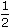# Aptitude - Logarithm - Discussion

### Discussion :: Logarithm - General Questions (Q.No.3)

3.

 log 8 is equal to: log 8

[A].
 1 8
[B].
 1 4
[C].
 1 2
[D].
 1 8

Explanation:

 log 8 = log (8)1/2 =log 8 = 1 . log 8 log 8 log 8 2

 Desmond Akatugba said: (Apr 14, 2011) pls explain further i dont really understand this

 Shalini said: (Sep 3, 2011) If given log m raise to the power n then power comes before log. (i.e) we can write it as : n log m.

 Gourav said: (Sep 28, 2013) Property of log is used i.e log m raise to power n miss @Shalimi is right. Work on some more problem on log like change of base formula which is very high end.

 Jayati said: (Oct 16, 2013) Can we cut log 8 from the denominator and numerator? referring to last step.

 Ramme said: (Sep 5, 2014) Why we can't put like this log 8 = log root(8) * log root(8) ?

 Ruhul Amin Ahmed said: (Oct 20, 2014) We know that, log(8)^1/2 = log8/2. So, answers is 1/2.

 Uday said: (Nov 5, 2014) 2 log 15/2-log 25/81+3 log 2/9 = log2 solve.

 Vennesa Bada said: (Sep 7, 2015) @Rahul Amin Ahmed. log 8^(1/2) = not log8/2.

 Keerthivasan said: (Sep 11, 2015) How to find log value?

 Kushal said: (Sep 24, 2015) What is antilog?

 Dishallar said: (Jun 29, 2016) Please help me with these logarithms given that log 3 = M. a) m+n as a logarithm of single number. b) log 9 in terms of m. c) log2 1/4 interms of m&n.

 Dishallar said: (Jun 29, 2016) Given that log 2= 0,3010 and 3 = 0,4771. Evaluate, a) Log 2, 25. b) Square root of log 60.

 Sindhuja said: (May 4, 2017) I am not getting this. Please explain it clearly.

 Shubham Kumar Singh said: (Jul 18, 2017) I am not getting this. Please explain me clearly.

 Abbhiranjan Singh said: (Jul 19, 2017) 2 concept goes here. 1. You can write root 8 in terms of power i.e 8^(1/2) then 1/2 is multiplied and now the term is (1/2)log8/log8 here many people think that log8/log8 cancel but this thing doesn't happen log cannot be cancelled. So we have to use the formula i.e, loga/logb i.e log(a-b) so here log( 8-8) gives the result log0 and value of log 0 is 1 so when 1 is multiplied with 1/2 it is 1/2.

 Satyabrata Panda said: (Apr 25, 2018) Thank you all for explaining it.

 Ananya Neogi said: (Jul 26, 2018) It is not clear to me. Please explain the answer in clear.

 Husain said: (Feb 9, 2019) =Log√8/Log8. =0.4515/0.9030, =0.5, =1/2.